# How to Stop Auto Calculation in Excel

This tutorial demonstrates how to stop Excel from calculating automatically and the difference between automatic and manual formula calculation.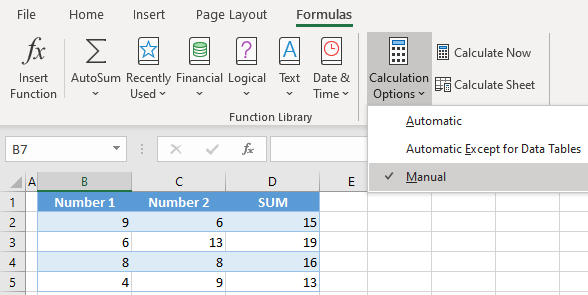## Stop Auto Calculation

By default, Excel recalculates every formula in a workbook whenever a cell is changed. This can slow down work, especially in complex files with a huge number of formulas. To speed up the process, you can stop auto-calculation of formulas by changing it to manual. This means that formulas won’t be calculated automatically on every change in a workbook. There are two ways to achieve this. The first one is, in the Ribbon, go to Formulas > Calculation Options > Manual.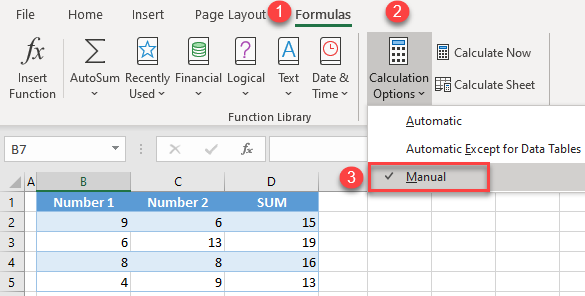Another way to switch to the manual calculation is to change Excel settings.

First, open Excel and select Options at the end of the menu.If you are already in an Excel workbook, in the Ribbon, select File > Options.

1. Now, select Formulas and go to the Calculation options section. Here you can find the Workbook calculation settings.
2. Select Manual.
3. Then click OK.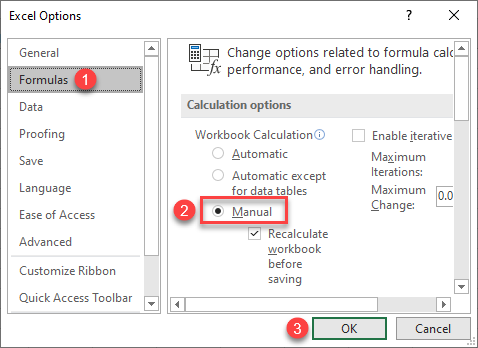## Manual Calculation: Calculate Now

To calculate formulas when auto-calculation is stopped, use Calculate Now in the Calculation Options. Let’s first see how data looks before calculating manually. For example, there are numbers in Columns A and B and the sum of those numbers in Column C.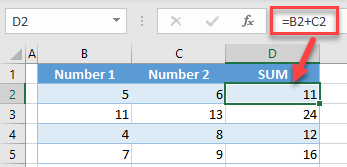If you change numbers in Columns A and B while auto-calculation is set to manual, you’ll see that sum in Column C does not update – no calculation is done.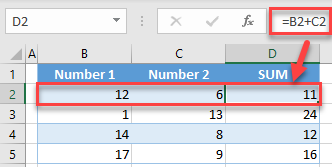So, when you’re ready to calculate all formulas, go to the (1) Formulas tab and click (2) Calculate Now.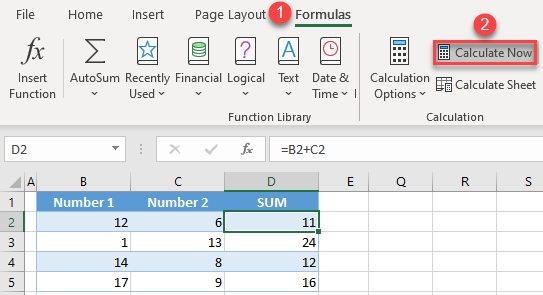Now all the formulas are updated, and the correct sums are calculated in Column C.